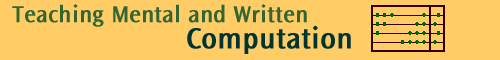Back to Multiply

Survey of mental methods for multiplication

This section shows some of the informal methods used by children to carry out multiplication, loosely classified according to the mathematical principles used. In practice, some mental methods used by children will have characteristics of more than one classification.

These methods are presented in a written form to make then easier to follow, but in practice they would be done mentally, or with some jottings on paper, organised in an idiosyncratic way.

Classifications Terminology

Front end multiplication (left to right)

Multiplication in stages (using factors)

Multiplication by rounding (using distributive property)

Multiplication by halving and doubling

Multiplying by special numbers

Other methods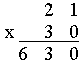multiplicand multiplier product

distributive property

Front end multiplication

When multiplying mentally, people generally like to do the bigger or important quantities first. This gives an estimate of the value expected. Such an approach is front end or left to right multiplication. Students using this method multiply the tens before the ones and hundreds before the tens and so on. They then add up these partial products to get the final result. This method is based on the distributive property of multiplication over addition.

Given below are some examples of front end multiplication. Note that there will be more than one possible way of presenting the written form as this is mainly used for mental computations.

 Mike Question: 23 x 8 I know that 23 is 20 + 3. So I multiply 20 by 8, and then 3 by 8. I now add 160 to 24 to get my answer of 184.Sam Question: 535 x 3 I know that with large numbers I can think of them in separate parts so 500 = 500 + 30 + 5 I like to start with the biggest number first. I can now easily add the parts together to get the answer.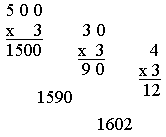Chris Question: 24 x 15 I am going to multiply 24 by 10 by first multiplying 20 by 10 and then 4 by 10. I am then multiply 24 by 5, by first multiplying 20 by 5 and then 4 by 5. I then add each of these results to get my final answer.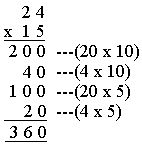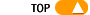Multiplication in stages

Some students simplify multiplication by multiplying by the factors one by one instead of the number itself. This method could help children learn multiplication tables. For instance, the 4xtables would be much easier if thought of as double the 2xtable, or that x6 is double of x3. Here are some examples to illustrate some of the procedures using this principle.

 Sarah Question: 25 x 6 I know that the factors of 6 are 2 and 3 which are both numbers I can easily multiply by. So I multiply the 25 by 2 first and then the answer by 3.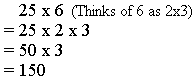Nick Question: 25 x 6 The factors of 25 are 5 and 5. If I multiply 5 by 6 I get 30. To multiply by 30 I can multiply by 3 and then 10.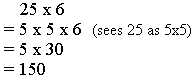David Question: 12 x 40 This method is often used when multiplication involves multiples of 10 as illustrated by David's method.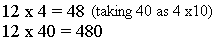Multiplication by rounding

Children usually use this method in multiplication problems where one of the numbers is close to a multiple of 10. Sometimes they use it for numbers nearby a known fact. Such a number is then rounded to the nearest ten or the known fact and then adjusted by addition or subtraction. Thus this method again uses the distributive property.

 Jason Question: 99 x 5 I notice that 99 is close to 100 which is number I can easily multiply by. I times 100 by 5 and 1 by five. Since 100 fives is 500, 99 fives will be 5 less.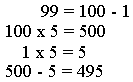Julia Question: 25 x 7 Four 25's make 100, so seven 25's is 100 + 25+25 + 25, total 175. Julia Question: 26 x 7 I already know 25 sevens, so I can use this.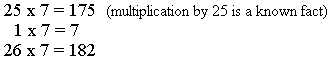Indira Question: 198 x 3 This is a difficult sum but I notice that 198 is close to 200. 200 times 3 is easy for me to solve. Since 200 threes is 600, 198 threes must be 2 threes less, so 600 minus 6.Multiplication by halving and doubling

Another method adopted by students is to double one factor and halve the other which leaves the product unaltered. This may be extended to four times one factor and quarter the other, or eight times one factor and eighth the other depending on the question. This method is actually a special and very common case of multiplication in stages.

 Andrew Question: 8 x 15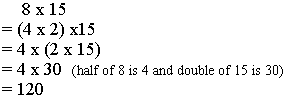Luke Question: 16 x 25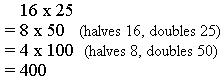Alan Question: 51 x 16 51 x 16 is difficult to solve,but I notice that I have two choices. 51 is close to 50 and 16 is easily halved to give 8. Halving is probably easier. I double 51 and halve 8 giving 102 x 8 This is now easily solved by adding 8 x 100 and 8 x 2.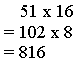This gives a quick method for multiplying by 25. Divide by 4 and add two zeroes.

Similarly to multiply by 50, divide by 2 and add two zeroes, to multiply by 125, divide by 8 and add three zeroes.

Encouraging students to look for patterns in multiplication and finding quick methods such as those above will help develop their mental multiplication skills and number sense.Multiplying by special numbers

Many students will enjoy working out special methods for special numbers. Here are some examples worth remembering. Classes should talk about WHEN and WHY these shortcuts work.

 Shortcuts Examples To multiply by 25, multiply by 100 and divide by 4 (because 25 is 100 divided by 4) 36 x 25 is 3600 divided by 4, so the answer is 900. To multiply by 125, multiply by 1000 and divide by 8 (because 125 is 1000 divided by 8). 56 x 125 is 56000 divided by 8, so the answer is 7000. To multiply by 9, multiply by 10 and subtract the number (because 9 is 10 minus 1). 123 x 9 is 1230 subtract 123, which is 1230 subtract 100 (so 1130), then subtract 20 (so 1110) then subtract 3 (so 1107) To multiply by 4, double twice (because 4 is 2 by 2). 65 x 4 is done by doubling 65 (get 130) and doubling that (get 260) To multiply by 11, add adjacent digits, because 11 is 10 + 1 62 multiplied by 11 is 682 (write down the 6, add the 6 and 2 and write down the 8, write down the 2) 6352 x 11 is 69872 (write down the 6, add 6 &3, write down 9, add 3 & 5, write down 8, add 5 & 2, write down 7, write down the 2) (Be careful: check what happens when the sums go over 10) Make up some shortcuts of your ownOther methods to suit specific questions

Apart from the methods classified above, children devise short cuts to suit the question at hand. Such methods often combine more than one of the above methods.

 Brendan Brendan uses repeated addition for 35 x 3. However for the 32 x 45 he uses halving and doubling followed by front end multiplication. Good mental calculators use a variety of methods, depending on the actual numbers in the question. The multiplier (3) is quite small so repeated addition can be easily used. Question: 35 x 3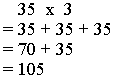Question: 32 x 45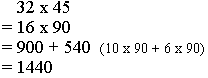Anna Anna uses her knowledge of algebra: she knows the "difference of two squares", a number pattern which she can express as follows. Question: 8 x 12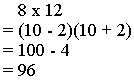More examples of this method: 7 x 13 = (10 - 3) (10 + 3) = 10 x 10 - 3x3 = 100 - 9 = 91 24 x 16 = (20 - 4) (20 + 4) = 20 x 20 - 4x4 = 400 - 16 = 384

 Monica Monica has combined halving and doubling and front end multiplication. It is clear that she knows her number principles and multiplication facts well but goes to great lengths to avoid the actual written algorithm. Although students' own methods should be encouraged, it is also important to teach students an efficient algorithm. As Monica's example show, although invented methods can give the correct answer, they may be more time consuming. 26 x 84 is difficult to solve. If I halve 26 to get a smaller number this will help. To multiply by 13, I will think of it as 10 plus 3. Using front end multiplication the first part is solved 10 x 168 =1680 168 is then seen as 100 + 60 + 8, which are each easily multiplied by 3. The separate products can now be added. Question: 26 x 84